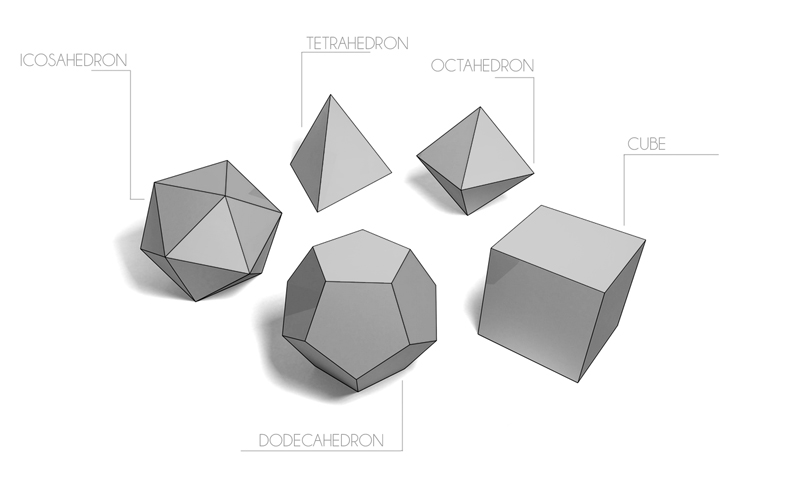# Platonic ResistanceYou have each of the five Platonic solids, with edges made out of $1\Omega$ resistors. For which solid can you pick two vertices that have an effective resistance between them that is greater than $1\Omega$?

Definitions:

• Tetrahedron is a polyhedron with 4 sides.

• Octahedron is a polyhedron with 8 flat faces.

• Dodecahedron is a polyhedron with 12 flat faces.

• Icosahedron is a polyhedron with 20 flat faces.

• Oblate spheroid is a quadric surface obtained by rotating an ellipse about its minor axis.

Clarification: "All of these" and "None of these" refer only to the geometrical answers not to each other!

×